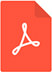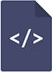# The numerical solution of the navier-stokes equations in nuclear shock wave propagationPDF Full Text

# Abstract

In this paper, we will consider Navier-Stokes problem and it’s interpretation by hyperbolic waves, focusing on wave propagation. We will begin with solution for linear waves, then present  problem  for  non-linear  waves.  Later  we  will  derive  for  numerical  solution  using  PDE`s. We can conclude that although the numeric solution for partial derivative equations can give a correct result, it is not always describing a physical phenomenon.

# Keywords

navier-stokes problem, hyperbolic waves, wave propagation, linear wave, non-linear waves